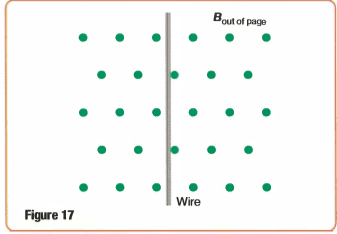# Direction of Electric field (EM induction)

## Homework Statement

In which direction would the electric field in the wire point if the wire were pulled to the right?Right hand rule
F=qvXB

## The Attempt at a Solution

My answer is downward. I am confused whether I should take the moving charges as positive or negative since this would determine the direction of the current.
I know that the direction of the current is the same as the electric field. (for positive)
Thanks!

#### Attachments

cnh1995
Homework Helper
Gold Member
I am confused whether I should take the moving charges as positive or negative since this would determine the direction of the current.
Which particles are mobile? Electrons or protons?

Electrons. But we take the convention of moving "positive charges"

cnh1995
Homework Helper
Gold Member
There is no "current" in this wire as there is no complete circuit. Protons and electrons both are moving towards right as the wire is dragged. What is the magnitude and direction of the forces acting on both the particles? What will happen because of these forces?